Search

Determinant Word ProblemsThere are some tricks you can do with determinants, and these tend to be turned into word problems.

• A triangle has vertices as (1, 2), (3, 4), and (2, 3). Find the area of the triangle.
• I could try to work from a drawing of the triangle, but this can get very complicated. Instead, I put the vertices of the triangle into a determinant, with the x-values being the first column, the corresponding y-values being the second column, and the third column all filled with 1's, like this: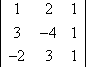Remember that the area formula for a triangle with base b and height h is A = ½ bh. So I need the ½ here, too. Evaluating:   Copyright © Elizabeth Stapel 2004-2011 All Rights Reserved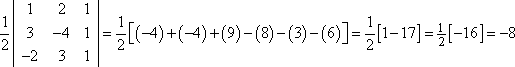Whoa! A negative area? Is that possible? No. So is the formula wrong? Sort of. Remember that a determinant can be negative, while areas (and absolute values) cannot. To account for this, the formula is actually "± ½ D", where I chose the "+" or the "" so that I get a positive answer. Then: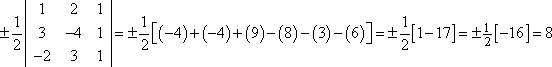Then the area of the triangle is 8 square units.

Does it matter in which order you list the points in the determinant? No: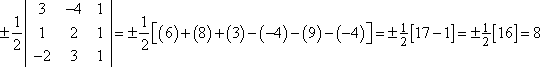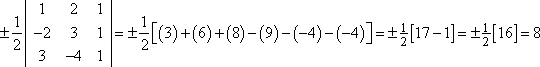The order of the points in the determinant doesn't matter because changing the order of the rows in a determinant doesn't change its value, except maybe for changing the sign.

• A parallelogram has vertices at (3, 4), (1, 2), (1, 3), and (3, 3). Find its area.
• A triangle is half of a parallelogram, so the area of a triangle is half that of a parallelogram. Then the formula for the area of a parallelogram is just the formula for the area of a triangle, but without the "½". But which three points do I plug into the formula? I've got three to choose from, and it turns out that it really doesn't matter which three I choose:

 using the points (3, 4), (1, 2) and (1, 3):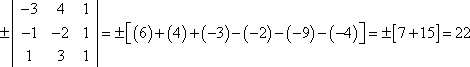using the points (3, 4), (1, 3) and (3, 3):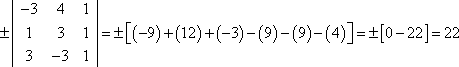using the points (1, 2), (1, 3) and (3, 3):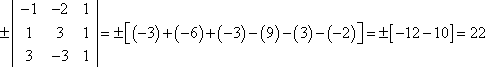using the points (3, 4), (1, 2) and (3, 3):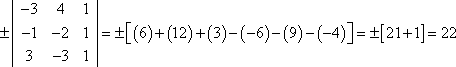Then the area of the parallelogram is 22 square units.

Note that, in the second and third cases, I had to change the sign to get a positive area. But in all cases, I got the same-sized value.

• A line passes through the point (2, 3) and (3, 1). Find its equation.
• Normally, I would find the slope, pick one of the points, and plug the slope and the point's coordinates into the slope-intercept form to find the line's equation. But I can use a determinant to find the same thing. I set up a determinant with an x-column, and y-column, and an all-ones column, and set it equal to zero, like this: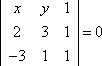Then I evaluate and simplify:

(x)(3)(1) + (y)(1)(3) + (1)(2)(1)  (3)(3)(1)  (1)(1)(x)  (1)(2)(y) = 0
3x  3y + 2 + 9  x  2y = 0

2x  5y + 11 = 0

The line has equation 2x  5y = 11.

(The format for the answer to this type of exercise will vary from text to text, so make a note of how your book does it, and match that format.)

The last exercise above shows why you will need to be able to evaluate determinants by hand: your teacher can give you an exercise like this that you can't solve with your calculator. So make sure you practice enough to learn the process.